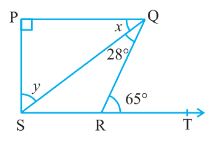Q

# In Fig. 6.43, if PQ ⊥ PS, PQ || SR, ∠ SQR = 28° and ∠ QRT = 65°, then find the values of x and y.

5. In Fig. 6.43, if PQ PS, PQ SR, SQR = 28° and QRT = 65°, then find the values of x and y.ViewsWe have,
PQ PS, PQ || SR, SQR = 28° and QRT = 65°
Now, In  QRS, the side SR produced to T and PQ || RS
therefore, QRT =  =
So,

Also, QRT = RSQ + SQR (By exterior angle property of a triangle)
Therefore, RSQ = QRT - SQR

Now, in  PQS,
P + PQS + PSQ =

Exams
Articles
Questions